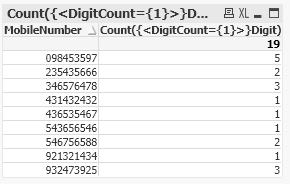# New to Qlik Sense

If you’re new to Qlik Sense, start with this Discussion Board and get up-to-speed quickly.

Announcements
Our May 2021 end-to-end product release from Data Integration to Data Analytics is out! READ DETAILS
cancel
Showing results for
Did you mean:Contributor

## Unique count

Hello,

How do I find the number of unique values from the column below and have a column that displays that?

Assignment:
[
MobileNumber
921321434
124321432
431432432
543656546
932473925
098453597
346576478
436535467
546756588
235435666
];

Exit Script;

Desired output is something like this

Mobilenumber  Uniquecount

921321434            1

932473925            3

and so on.

Im trying to do this with Count ( Distinct Mobilenumber)) but I guess I dont have the syntax right or maybe the logic itself is wrong. Id appreciate an answer with the correct syntax as well.

Thanks

1 Solution

Accepted SolutionsMVP

One solution could be like:

``````Assignment:
MobileNumber, Digit,
Count(Digit) as DigitCount
Group By MobileNumber, Digit;
Join
*,
Mid(MobileNumber,IterNo(),1) as Digit
While IterNo()<=Len(MobileNumber);

[
MobileNumber
921321434
124321432
431432432
543656546
932473925
098453597
346576478
436535467
546756588
235435666
];``````6 RepliesPartner

Hi,

The Distinct in correct, but this is the difference:

``````// This gives you always 1 for each mobile number. Because you are counting the distinct ones
Count(Distinct Mobilenumber)

// This gives you the amount of mobile numbers if your dimension is Mobilenumber. This is what you are showing in your example.
Count(Mobilenumber)``````

Jordy

Climber

Work smarter, not harderContributor
Author

The aim is to get the number of unique values in each mobilenumber. For example:

921243444 has '9' '1' and '3' as unique values since they only appear once in the above mobile number. So the count would be 3.Specialist II

Hi

Not very nice but try this using fonction substringcount

count(if(SubStringCount(MobileNumber,9)=1,1))+
count(if(SubStringCount(MobileNumber,8)=1,1))+
count(if(SubStringCount(MobileNumber,7)=1,1))+
count(if(SubStringCount(MobileNumber,6)=1,1))+
count(if(SubStringCount(MobileNumber,5)=1,1))+
count(if(SubStringCount(MobileNumber,4)=1,1))+
count(if(SubStringCount(MobileNumber,3)=1,1))+
count(if(SubStringCount(MobileNumber,2)=1,1))+
count(if(SubStringCount(MobileNumber,1)=1,1))+
count(if(SubStringCount(MobileNumber,0)=1,1))MVP

Use the phone number  as a dimension, and this expression:

-((SubStringCount(PhoneNumber, '0') = 1) +
(SubStringCount(PhoneNumber, '1') = 1) +
(SubStringCount(PhoneNumber, '2') = 1) +
(SubStringCount(PhoneNumber, '3') = 1) +
(SubStringCount(PhoneNumber, '4') = 1) +
(SubStringCount(PhoneNumber, '5') = 1) +
(SubStringCount(PhoneNumber, '6') = 1) +
(SubStringCount(PhoneNumber, '7') = 1) +
(SubStringCount(PhoneNumber, '8') = 1) +
(SubStringCount(PhoneNumber, '9') = 1))

Change PhoneNumber to the correct field name containing the phone number

Logic will get you from a to b. Imagination will take you everywhere. - A EinsteinMVP

One solution could be like:

``````Assignment:
MobileNumber, Digit,
Count(Digit) as DigitCount
Group By MobileNumber, Digit;
Join
*,
Mid(MobileNumber,IterNo(),1) as Digit
While IterNo()<=Len(MobileNumber);

[
MobileNumber
921321434
124321432
431432432
543656546
932473925
098453597
346576478
436535467
546756588
235435666
];``````Contributor
Author

This works

Thank youTags